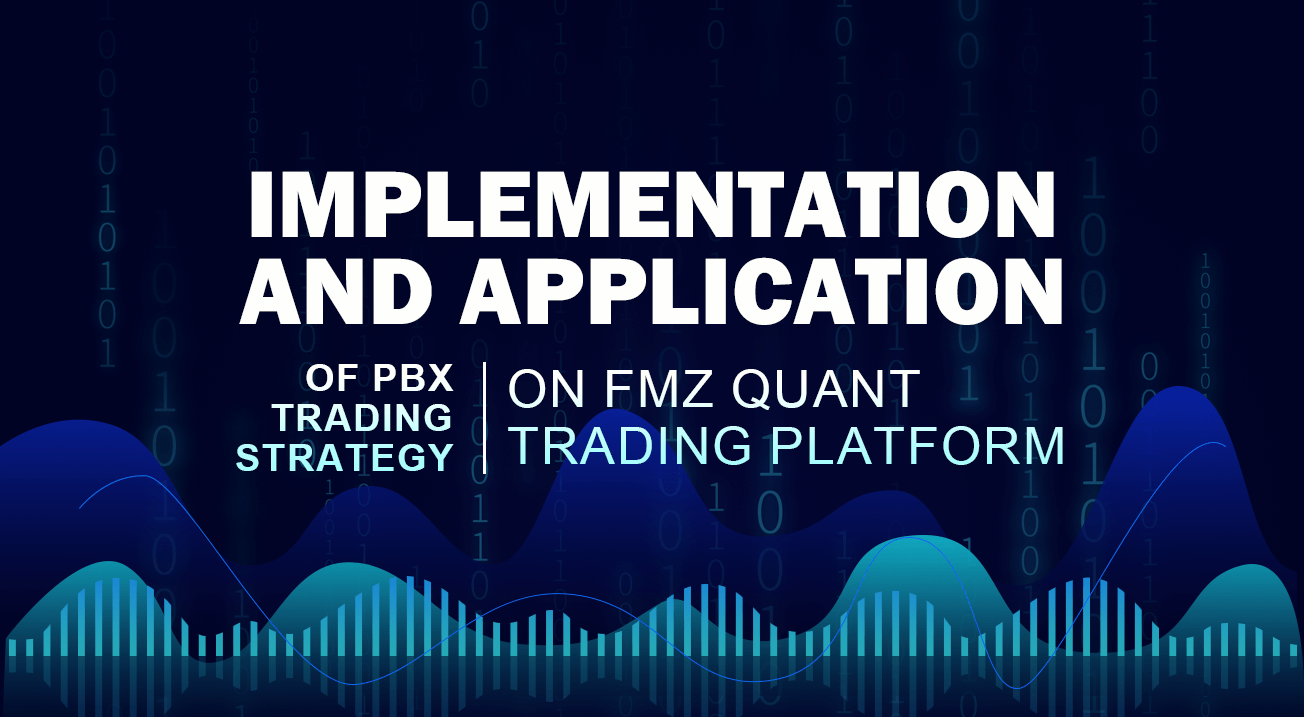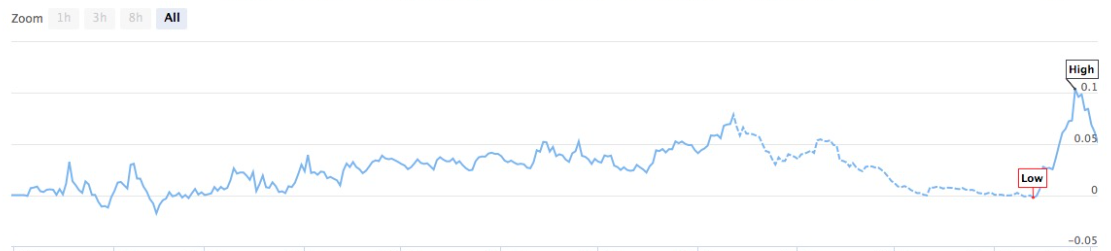# Implementation and application of PBX trading strategy on FMZ Quant Trading platform

Author: Lydia, Created: 2023-01-18 10:31:11, Updated: 2023-09-20 11:13:19## Implementation and application of PBX trading strategy on FMZ Quant Trading platform

### Definition of PBX

PBX refers to the moving average drawn by the dissimilar moving average (MACD) index, which is used to generate buy and sell signals in securities. The PBX or signal line is the moving average (EMA) of the nine periods of the MACD indicator line used by traders to predict the future price trend. Although the nine-period EMA is the default setting of the PBX, traders can adjust the length of the EMA to adapt to their trading targets and strategies.

### The significance of PBX

The PBX provides technical insights on when to open long or short positions. When the PBX exceeds or falls below the EMA indicator, traders will enter the market and close positions based on this. When EMA exceeds the PBX, it generates a buy signal, indicating that traders should buy and open positions. On the contrary, if EMA falls below the PBX, it indicates a bearish trend, and traders should go short.

### Benefits of using PBX

Quick response: The PBX helps to detect the trend reversal as soon as possible, which makes it a particularly useful tool for short-term traders. Since the PBX adopts nine periods of EMA, it can respond to price changes quickly. This helps offset the lagging nature of the indicator.

Use PBX to systematize transaction decision-making. Traders can stay in one direction until the PBX crosses the MACD in the opposite direction. For example, if the EMA keeps a long position when it crosses the PBX, the trader can only do long trading in these positions until the MACD crosses below the PBX. Entering and exiting the market through signals generated by the PBX will prevent traders from making second guesses or making random decisions.

In a volatile market, the PBX will often cross the EMA and generate many false trading signals. To avoid this situation, traders can try to add other technical indicators to support it. For example, when EMA crosses the PBX, it requires the volume index (MFI) to be oversold. Another example is to use a random oscillator with a PBX. Traders need to confirm that the K-line crosses the D-line before the MACD exceeds the PBX.

### Implement a PBX strategy

Now that we know the basic definition and principle of the PBX, let’s implement this strategy on the FMZ Quant Trading platform. The programming language is still the MyLanguage, which is easy to understand. Readers can extend or improve according to the following code.

• Strategy name: PBX PUBU trading strategy
• Data period: 15M
• Support: commodity futures, digital currency• Main chart
``````PBX 1, formula: PUBU1^^(EMA(C,N1)+EMA(C,N12)+EMA(C,N14))/3;
PBX 2, formula: PUBU2^^(EMA(C,N2)+EMA(C,N22)+EMA(C,N24))/3;
PBX 3, formula: PUBU3^^(EMA(C,N3)+EMA(C,N32)+EMA(C,N34))/3;
``````

MyLanguage source code:

``````// Indicator
PUBU1^^(EMA(C,N1)+EMA(C,N1*2)+EMA(C,N1*4))/3;
PUBU2^^(EMA(C,N2)+EMA(C,N2*2)+EMA(C,N2*4))/3;
PUBU3^^(EMA(C,N3)+EMA(C,N3*2)+EMA(C,N3*4))/3;

BKVOL=0 AND BARPOS>N3 AND C>PUBU1 AND PUBU1>PUBU2 AND PUBU2>PUBU3,BPK;
SKVOL=0 AND BARPOS>N3 AND C<PUBU1 AND PUBU1<PUBU2 AND PUBU2<PUBU3,SPK;

C<PUBU3,SP(BKVOL);
C>PUBU3,BP(SKVOL);
C<PUBU2 AND PUBU1<PUBU2 AND C>BKPRICE,SP(BKVOL);
C>PUBU2 AND PUBU1>PUBU2 AND C<SKPRICE,BP(SKVOL);
AUTOFILTER;
``````

For the strategy source code, please refer to: https://www.fmz.com/strategy/128420.

Related

More